Question

A.Zero Coupon Bonds A 7 year maturity zero coupon corporate bond has an 8% promised yield. The bond's price should equal

 B.The Fishing Pier has 6.40 percent, semi-annual bonds outstanding that mature in 12 years. The bonds have a face value of \$1,000 and a market value of \$1,027. What is the yield to maturity?

C.Bond Yields Find the promised yield to maturity for a 7% coupon, \$1,000 par 20 year bond selling at \$1115.00. The bond makes semiannual coupon payments.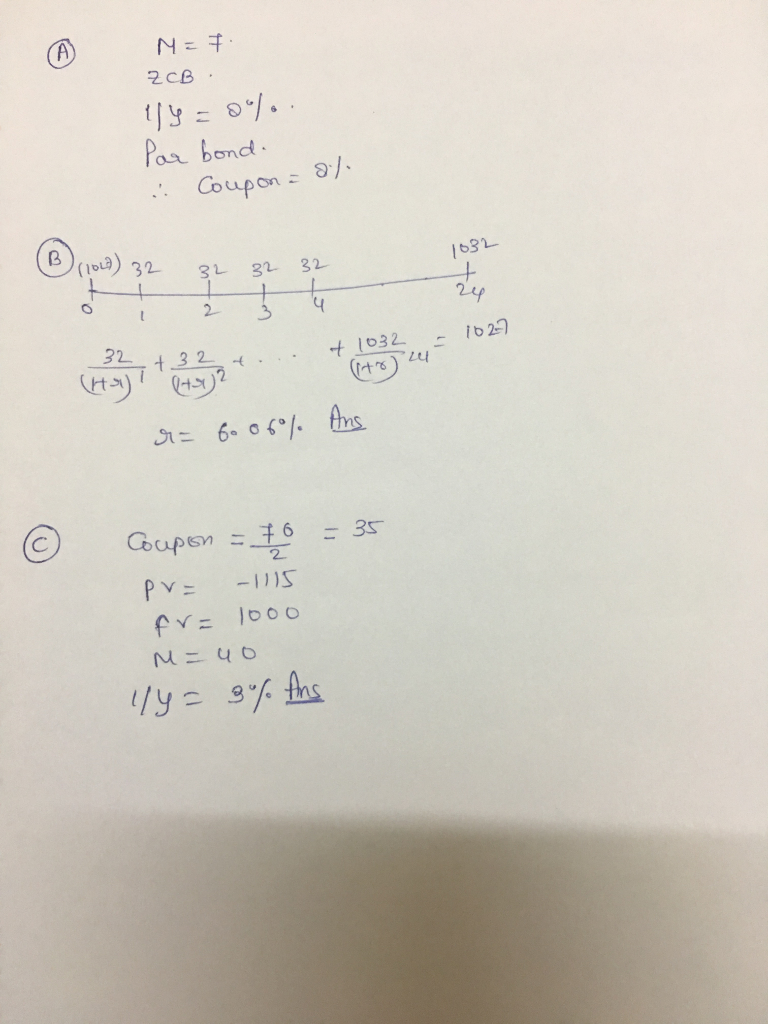SOLUTION :

A.

Let us assume face value of bod = 100(\$)

So, price of bond = 100/(1 + 0.08)^7 = 58.35 ( % of face value)

Bond price is quoted as % of face value.

B.

Use online calculator for YTM : ref. https://dqydj.com/bond-yield-to-maturity-calculator/

Enter :

Current price (\$) = 1027

Face value (\$) = 1000

Years to maturity = 12

Coupon rate (%) = 6.40

Coupon payments frequency = 2

Press compute , we get YTM = 6.080 % (ANSWER).

C

Use online calculator for YTM : ref. https://dqydj.com/bond-yield-to-maturity-calculator/

Enter :

Current price (\$) = 1115

Face value (\$) = 1000

Years to maturity = 20

Coupon rate (%) = 7

Coupon payments frequency = 2

Press compute , we get YTM = 6.005 % (ANSWER).

answered by: Tulsiram Garg

#### Earn Coins

Coins can be redeemed for fabulous gifts.

Similar Homework Help Questions
• ### 6. Bond Valuation A BBB-rated corporate bond has a yield to maturity of 9%. AU.S. Treasury...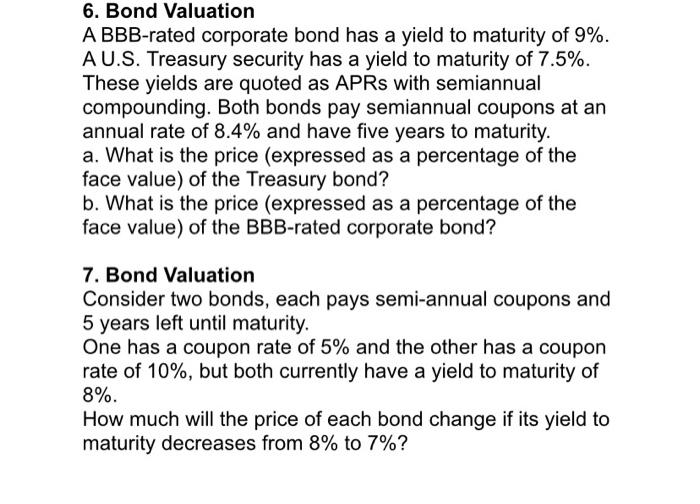6. Bond Valuation A BBB-rated corporate bond has a yield to maturity of 9%. AU.S. Treasury security has a yield to maturity of 7.5% These yields are quoted as APRS with semiannual compounding. Both bonds pay semiannual coupons at an annual rate of 8.4% and have five years to maturity a. What is the price (expressed as a percentage of the face value) of the Treasury bond? b. What is the price (expressed as a percentage of the face value)...

• ### An 8% coupon bond with 3 years to maturity has a yield of 7%. Assume that...

An 8% coupon bond with 3 years to maturity has a yield of 7%. Assume that coupon is paid semi-annually and face value is \$1,000. (a) Calculate the price of the bond. (Keep 2 decimal places, e.g. 90.12)   (b) Calculate the duration of the bond. (Keep 4 decimal places, e.g. 5.1234)   (c) Calculate this bond's modified duration. (Keep 4 decimal places, e.g. 5.1234)   (d) Assume that the bond's yield to maturity increases from 7% to 7.2%, estimate the new price...

• ### A​ BBB-rated corporate bond has a yield to maturity of 6.4%. A U.S. treasury security has a yield to maturity of 4.4%. T...

A​ BBB-rated corporate bond has a yield to maturity of 6.4%. A U.S. treasury security has a yield to maturity of 4.4%. These yields are quoted as APRs with semiannual compounding. Both bonds pay​ semi-annual coupons at a rate of 4.7% and have five years to maturity. a. What is the price​ (expressed as a percentage of the face​ value) of the treasury​ bond? b. What is the price​ (expressed as a percentage of the face​ value) of the​ BBB-rated...

• ### 7.) The yield to maturity of a \$ 1,000 bond with a 6.7 % coupon​ rate,...

7.) The yield to maturity of a \$ 1,000 bond with a 6.7 % coupon​ rate, semiannual​ coupons, and two years to maturity is 7.7 % ​APR, compounded semiannually. What is its​ price? The price of the bond is ​\$ __ 8.) Suppose a​ ten-year, \$ 1 comma 000 bond with an 8.4 % coupon rate and semiannual coupons is trading for \$ 1 comma 035.89. a. What is the​ bond's yield to maturity​ (expressed as an APR with semiannual​...

• ### A​ BBB-rated corporate bond has a yield to maturity of 6.3 % A U.S. treasury security...

A​ BBB-rated corporate bond has a yield to maturity of 6.3 % A U.S. treasury security has a yield to maturity of 4.3 % These yields are quoted as APRs with semiannual compounding. Both bonds pay​ semi-annual coupons at a rate of 5.0 % and have five years to maturity.     a. What is the price​ (expressed as a percentage of the face​ value) of the treasury​ bond? b. What is the price​ (expressed as a percentage of the face​ value)...

• ### 19. A bond has 8 years to maturity, a 7 percent coupon, a \$1,000 face value,...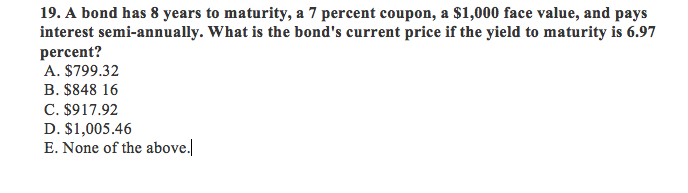19. A bond has 8 years to maturity, a 7 percent coupon, a \$1,000 face value, and pays interest semi-annually. What is the bond's current price if the yield to maturity is 6.97 percent? A. \$799.32 B. \$848 16 C. \$917.92 D. \$1,005.46 E. None of the above.l 19. A bond has 8 years to maturity, a 7 percent coupon, a \$1,000 face value, and pays interest semi-annually. What is the bond's current price if the yield to maturity is...

• ### • Comedy Corporation's bonds have a 15-year maturity, a 7% annual coupon paid with semiannual frequency,...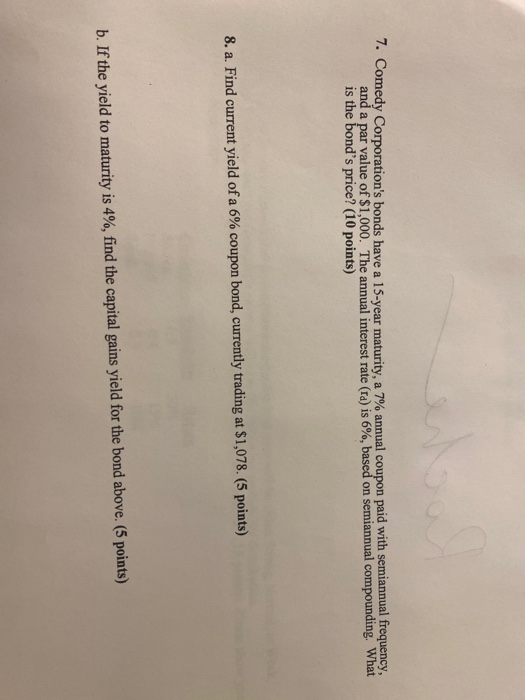• Comedy Corporation's bonds have a 15-year maturity, a 7% annual coupon paid with semiannual frequency, and a par value of \$1,000. The annual interest rate (ra) is 6%, based on semiannual compounding, What is the bond's price? (10 points) 8. a. Find current yield of a 6% coupon bond, currently trading at \$1,078. (5 points) b. If the yield to maturity is 4%, find the capital gains yield for the bond above. (5 points)

• ### A BBB-rated corporate bond has a yield to maturity of 7.9%. AU.S. Treasury security has a...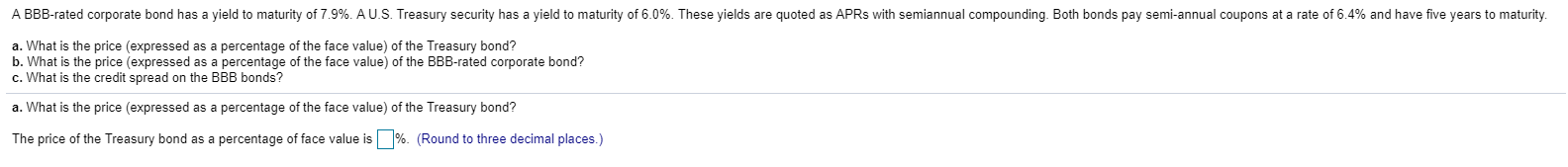A BBB-rated corporate bond has a yield to maturity of 7.9%. AU.S. Treasury security has a yield to maturity of 6.0%. These yields are quoted as APRs with semiannual compounding. Both bonds pay semi-annual coupons at a rate of 6.4% and have five years to maturity. a. What is the price (expressed as a percentage of the face value) of the Treasury bond? b. What is the price (expressed as a percentage of the face value) of the BBB-rated corporate...

• ### Oil Well Supply offers 7.4 percent coupon bonds with semiannual payments and a yield to maturity...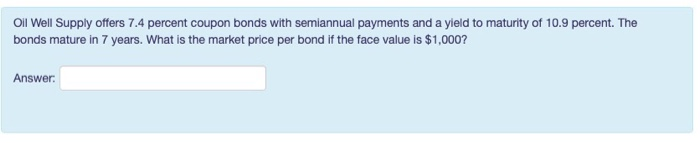Oil Well Supply offers 7.4 percent coupon bonds with semiannual payments and a yield to maturity of 10.9 percent. The bonds mature in 7 years. What is the market price per bond if the face value is \$1,000? Answer The outstanding bonds of The River Front Ferry carry a 8.1 percent coupon. The bonds have a face value of \$1,000, 10 years to maturity, and are currently quoted at \$1215.19. What is the current yield on these bonds? Provide your...

• ### 1. The following table provides zero coupon bond yields. Maturity Bond equivalent yield 6 months 6%...

1. The following table provides zero coupon bond yields. Maturity Bond equivalent yield 6 months 6% 1 year 8% A 12% coupon bond with coupons paid semiannually matures in one year. The par value of the bond is \$1,000. What is the price of this bond? [First identify the cash flows.] A. \$1,030 B. \$1,032 C. \$1,034 D. \$1,038 2. The following are the prices of zero coupon bonds. Par value is \$1,000 in each case. Maturity Price 6 months...